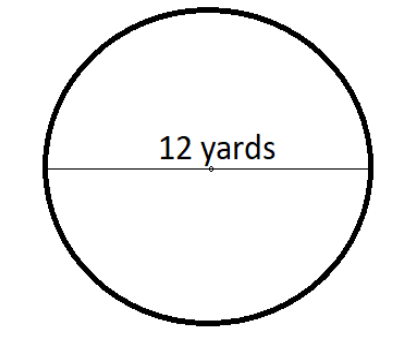Courses
Courses for Kids
Free study material
Offline Centres
MoreLast updated date: 01st Dec 2023
Total views: 279k
Views today: 5.79k

# What is the circumference of a circle whose diameter is $12$ yards?Verified
279k+ views
Hint: In the given question, we have to find the circumference which can be found out by using the formula, $C = \pi d$. We are already provided with the diameter so we just have to apply the formula. Circumference is basically the boundary of the circle.

Circumference refers to the distance around a circle or some other curved geometrical shape. It's the one-dimensional linear measurement of any two-dimensional circular surface's boundary. It follows the same principle behind finding the perimeter of any polygon, which is why calculating the circumference of a circle is also known as the perimeter of a circle. A circle is defined as a shape with all the points are equidistant from a point at the centre.
It is usually measured in units, such as centimetre, metres, yards etc.
We can calculate the circumference with help of the following formula:
$C = \pi d$
Where
$C =$ Circumference of the circle
$d =$ Diameter of the circle
$\pi = \dfrac{{22}}{7}\,or\,3.14 =$ Constant
Since diameter of the circle is twice the radius ($r$), the formula can also be written as-
$C = \pi 2r$
We are given $d = 12$. It is shown in diagram as follows:Thus, applying the formula, circumference of the circle will be-
$C = 3.14 \times 12$
$C = 37.68$ yards.
Hence the circumference of a circle whose diameter is $12$ yards is $37.68$ yards.
So, the correct answer is “$37.68$ yards.”.

Note: Circumference should not be confused with the area of the circle. Area is the space occupied by the circle whereas circumference is only the boundary of the circle. The formula to find the area of the circle is $A = \pi {r^2}$.